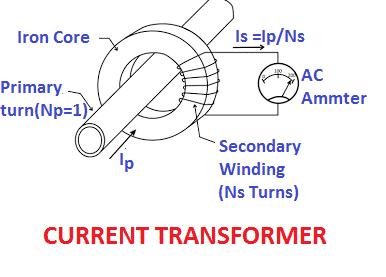# Instrument Safety Factor of Current TransformerThe current transformer is used for measuring the current.The specifications of the measuring class CT is as given below. The Instrument Safety Factor (ISF) is very important parameter for measuring class CT.
 CTR CT Terminal CT Burden Accuracy Class CT ISF 200/5 1S1-1S2 10 0.5 10

The secondary of the current transformer is connected to ampere meter, energy meter or protection relay. The current measuring instrument has its current measuring range. It also has overload capacity for shorter period of time.

If the current flows through the measuring instrument is more than its overload current capacity the instrument is apt to fail. Lets take an example-The overload current capacity of the measuring instrument of 5 ampere rating may be of 50 ampere for 3 sec or 5 sec. Why 50-ampere current should flow through an ammeter if normal circuit current is 5 ampere.

The electrical components of the electrical network is designed for carrying the fault current for definite time.The breaker rated for 1600 ampere current can carry fault current of 21 kA for 1 second or 3 seconds.Therefore, the measuring instruments are also designed to carry the increased fault current for definite time, The maximum current which can flow through the measuring instrument depends on what is the overload current capacity of the measuring instrument.

When CT is connected to measuring instrument, the normal current flows through the CT. A 1000/5 ampere CT carry 5 ampere in its secondary if primary current is 1000 ampere, and CT operates at certain voltage on its magnetization curve. During fault the maximum current through the CT primary may be  order of 10 kA.

It means 1000/5 ampere CT will carry about 10 times more current in its primary during fault and secondary current is 5 x 10=50 ampere. If the instrument which has normal current rating 5 ampere and overload capacity 6 x In=30 ampere, the measuring instrument which  will certainly get failed if 50 ampere current flows through it.

Here comes the importance of current transformer safety factor. Under fault condition, the CT will get saturate and CT secondary voltage gets a limit and current transformer behaves as a open circuit and no current flows through its secondary.

Let us understand the security factor of current transformer with an example. A CT having CTR of 1000/5 and fault current is 10 kA. We assume that if current exceeds above 10 kA the CT will get saturated. The ratio of CT saturation  current to primary rated current is 10000/1000=10. This ratio is called the security factor of current transformer. The ammeter connected to 1000/5 ampere CT shall be protected provided the instrument overload capacity is 10 times of its nominal current.

Therefore, the instrument safety factor of CT must be calculated and accordingly the overload capacity of the instrument connected to CT must be chosen.

The instrument security factor(ISF) is defined for metering applications.

## How to Calculate ISF of CT?

Step 1

Calculate the fault current of the network for which CTs are to be installed. The fault current of the network depends on the installed capacity in MVA and percentage impedance of the electrical network. Assume symmetrical fault current of the electrical network is 20 kA.

Step 2

Select CTR of current transformer for particular application. Let CTR of current transformer is 2000/5. The CT must be capable to carry about 10 times of its rated primary current during fault of 20 kA. Above 10 times of the current the CT gets saturate and it behaves as open circuit. The maximum current capacity of CT in this case is 10 times of its nominal current carrying capacity and we can say instrument safety factor of current transformer is 10. The instrument safety factor of CT can be mathematically written as;From above example,### How to select overload capacity of  current measuring instrument?

If the secondary current of the CT is 50 ampere.The instrument safety factor of the CT  is defined for metering class CTs.

At the time of procurement of measuring instrument the Instrument safety factor of current transformer(ISF of CT) must be checked for particular application. The overload specifications of the ampere meter according to IEC 60050 and EN 60051 are as follows.

Nominal Range(In) – 0- 20 Amper

Overload                – 10 x In, 5 seconds

The meter can sustain current up to 200 ampere(10 times of the nominal current) for 5 seconds. It means the safety factor of the current transformer must be 10. If current flows through the CT secondary is above 200 ampere the CT will get saturated and the CT secondary current reduces to zero and thus it protects the ammeter.
1.